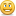• ##### #1, by brut69Saturday, 22. June 2013, 10:40 10 years ago
Hi,

I set a Value to be random between 1 and 10 and I want my script to get that value and print it
Value name 'sec'

I did this

------------
function start()

local action= getObject("Values[sec]")

if Values[sec]==1 then
print('1')
end
if Values[sec]==2 then
print('2')
end
if Values[sec]==3 then
print('3')
end
if Values[sec]==4 then
print('4')
if Values[sec]==5 then
print('5')
end
if Values[sec]==6 then
print('6')
end
if Values[sec]==7 then
print('7')
end
if Values[sec]==8 then
print('8')
end
if Values[sec]==9 then
print('9')
end
if Values[sec]==10 then
print('10')
end

end

----------------

For the life of me it doesn't work and I can't figure out why since this is so simple.
Any clues?

') = ' )

### brut69

#### 266 Posts

• ##### #3, by afrlmeSaturday, 22. June 2013, 13:19 10 years ago
it randomly seems to parse smilies in code blocks too.

``````-- let's set the initial value of sec variable
local sec = 0

-- let's create the function for checking random value of sec value
function checkRandVal()
sec = getObject("Values[sec]"):getInt(VValueInt)

if sec == 1 then
-- do some action!
elseif sec == 2 then
-- do some action!
elseif sec == 3 then
-- do some action!
elseif sec == 4 then
-- do some action!
elseif sec == 5 then
-- do some action!
elseif sec == 6 then
-- do some action!
elseif sec == 7 then
-- do some action!
elseif sec == 8 then
-- do some action!
elseif sec == 9 then
-- do some action!
elseif sec == 10 then
-- do some action!
end
print('value of sec =' .. sec)
end
``````

you don't actually need to use a value in the editor...
you could also create a variable with a random value.

``````-- let's set the initial value of sec variable
local sec = 0

-- let's create the function for checking random value of sec value
function checkRandVal()
sec = math.random(1,10)

if sec == 1 then
-- do some action!
elseif sec == 2 then
-- do some action!
elseif sec == 3 then
-- do some action!
elseif sec == 4 then
-- do some action!
elseif sec == 5 then
-- do some action!
elseif sec == 6 then
-- do some action!
elseif sec == 7 then
-- do some action!
elseif sec == 8 then
-- do some action!
elseif sec == 9 then
-- do some action!
elseif sec == 10 then
-- do some action!
end
print('value of sec =' .. sec)
end
``````

### afrlme

#### 7227 Posts

• ##### #4, by brut69Saturday, 22. June 2013, 13:31 10 years ago
Works perfect

Thank you

### brut69

#### 266 Posts

• ##### #5, by afrlmeSaturday, 22. June 2013, 14:25 10 years ago
welcomecouple things to take note of...

be careful when naming variables & functions: don't use names like start, end, action, run etc - in case they turn out to be actual Lua functions/commands already - use abbreviated versions instead.

Also make sure you don't use same names for functions/variables multiple times - could create issues if you have more than one instance that isn't local to x script or function.

using "elseif" is better than "else if" as you only need to add one "end" instead of one for each "if" used.

final note: when using the short getObject method rather than full path method, you have to make sure you don't have multiple instances of whatever you are trying to retrieve.
``````-- cleaner method: we are directly linking to the object condition/value or whatever
getObject("Scenes[scene_name].SceneObjects[object_name].SceneConditions[condition_name]"):getBool(VConditionValue)
-- or
getObject("Scenes[scene_name].SceneConditions[condition_name]"):getBool(VConditionValue)
-- etc...
``````